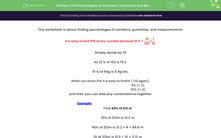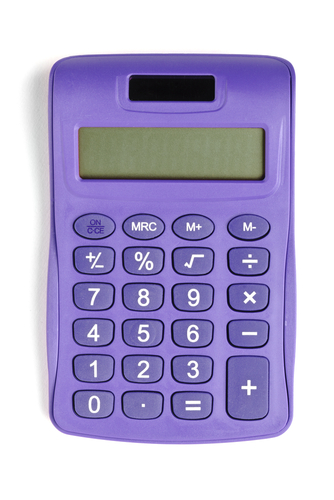# Find Percentages of Numbers, Quantities and Measurements

In this worksheet, students will find percentages of numbers, quantities and measurements.Key stage:  KS 3

Curriculum topic:   Number

Curriculum subtopic:   Define, Interpret and Compare Percentages

Popular topics:   Fractions worksheets, Percentages worksheets

Difficulty level:#### Worksheet Overview

This activity is about finding percentages of numbers, quantities, and measurements.

It is easy to find 10% of any number because 10% = 10/100 = 1/10

Simply divide by 10:

So 10% of 192 is 19.2

10% of 54 g is 5.4 g etc.

When you know 10%, it is easy to find 1% - simply divide by 10 again!

To find 2%, we can find 1% and multiply by 2.

To find 5%, we can find 10% and divide by 2.

To find 30%, we can find 10% and multiply by 3.

We can then add any combinations together!

Example

Find 43% of 212 m

10% of 212 m is 21.2 m

40% of 212 m is 21.2 × 4 = 84.8 m

1% of 212 m is 21.2 ÷ 10 = 2.12 m

3% of 212 m is 2.12 × 3 = 6.36 m

43% of 212 m = 40% + 3% = 84.8 + 6.36 = 91.16 m

You can work out percentages on a calculator either by using the % key, or by multiplying the number by the required percentage and dividing by 100.For this activity, we will be using the method above, so calculators allowed only for complicated divisions or multiplications!

Let's get started on the questions.

### What is EdPlace?

We're your National Curriculum aligned online education content provider helping each child succeed in English, maths and science from year 1 to GCSE. With an EdPlace account you’ll be able to track and measure progress, helping each child achieve their best. We build confidence and attainment by personalising each child’s learning at a level that suits them.

Get started#### Popular Maths topics

••••